# Difference between revisions of "De Moivre formula"

The formula expressing the rule for raising a complex number, expressed in trigonometric form \begin{equation} z = \rho(\cos\varphi + i\sin\varphi), \end{equation} to an $n$-th power. According to de Moivre's formula the modulus $\rho$ of the complex number is raised to that power and the argument $\varphi$ is multiplied by the exponent: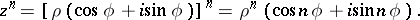The formula was found by A. de Moivre (1707), its modern notation was suggested by L. Euler (1748).

De Moivre's formula can be used to express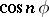and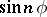in powers of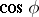and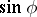: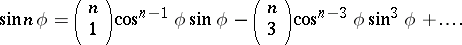Inversion of de Moivre's formula leads to a formula for extracting roots of a complex number: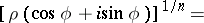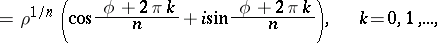which is also sometimes called de Moivre's formula.